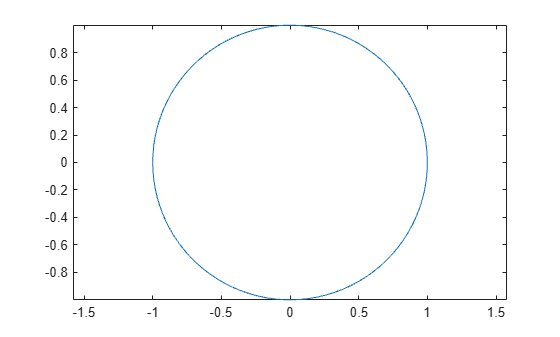# 对图形对象进行动画处理

```theta = linspace(-pi,pi); xc = cos(theta); yc = -sin(theta); plot(xc,yc); axis equal ``````xt = [-1 0 1 -1]; yt = [0 0 0 0]; hold on t = area(xt,yt); % initial flat triangle hold off for j = 1:length(theta)-10 xt(2) = xc(j); % determine new vertex value yt(2) = yc(j); t.XData = xt; % update data properties t.YData = yt; drawnow limitrate % display updates end ```